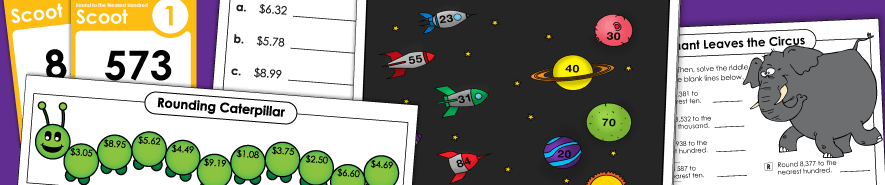# Rounding## Rounding Whole Numbers

Rounding to Nearest Ten

If you're teaching kids to round 2 and 3-digit numbers to the nearest ten, take a look at this page. It has a Scoot game, lots of worksheets, and rounding charts.
(examples: 75 rounds to 80; 167 rounds to 170)

Rounding to Nearest Hundred

On this worksheets, pupils will round numbers to the nearest hundred. There's also a section for rounding to nearest ten and hundred (mixed).
(examples: 345 rounds to 300; 1,677 rounds to 1,700)

Rounding to Nearest Thousand

This section has a few printables on rounding to the nearest thousand.
(example: 5,765 rounds to 6,000)

## Decimal and Money Rounding

Decimal Rounding

Round decimals to the nearest tenth, hundredth, thousandth, and/or whole number.
(example: 2.67 rounds to 3)

Money: Rounding to Nearest Dollar and Nearest Dime

Round money amounts to the nearest dollar. There's a math riddle activity, and practice worksheets.
(example: \$6.71 rounds to \$7.00)

Money: Next Dollar Up

Round each amount up to the next dollar to determine how many bills to give a cashier.
(example: \$4.12 rounds up to \$5.00)

Place Value

Super Teacher Worksheets has a HUGE collection of place value worksheets. Use these resources for teaching kids about comparing and ordering numbers, place value blocks, expanded notation, and digit values.